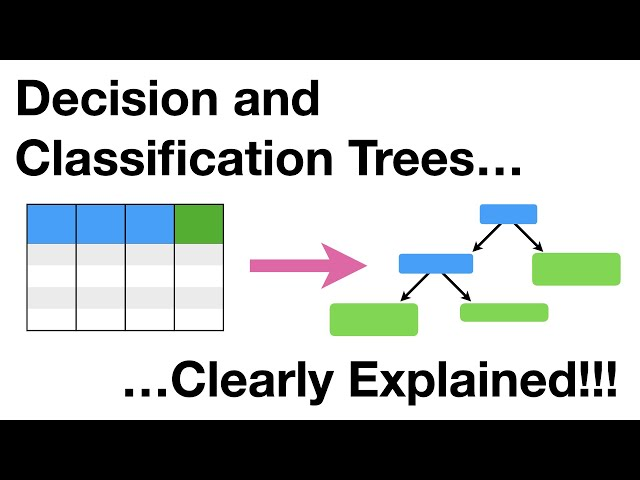# What is Decision Trees

##### Introduction to Decision Trees

Decision Trees are powerful tools used in machine learning for solving complex problems. They help in predicting an output for a given input by mapping it to a set of classified outputs. In simple terms, decision trees are a graphical representation of a set of decision rules that are used to make decisions based on the given input parameters. It is a predictive model that is used for both classification and regression problems.

A decision tree is a tree-like structure that breaks down a dataset into smaller and smaller subsets while at the same time, creating an associated decision tree. The final result is a tree with decision nodes and leaf nodes. The decision nodes represent the attributes or features that the dataset is split on, and the leaf nodes represent the decision or the output of the tree. Decision trees are easy to understand, interpret and implement which makes them a popular choice in machine learning and data science.

##### Key Concepts of Decision Trees

There are a few key concepts that are important to understand in order to effectively use decision trees.

• Tree Depth: This is the distance or the number of nodes from the root node to the leaf node. It is the maximum number of decisions that can be made in the tree. It is an important factor to consider while building decision trees as it can help avoid overfitting or underfitting the data.
• Information Gain: It is the amount of information that is gained by branching on a particular attribute. It is used to determine which attribute to split the dataset on. The attribute with the highest information gain is chosen to split the data.
• Entropy: It is a measure of the impurity of a dataset. A dataset with only one class or output has an entropy of 0, while a dataset with equal distribution among all classes has an entropy of 1. Entropy helps in determining the best split for a dataset.
• Gini Index: It is a measure of how often a randomly chosen element would be incorrectly classified if it were randomly classified according to the distribution of labels in a dataset. Gini Index is used to select the best split for a dataset.
##### Types of Decision Trees

There are two main types of decision trees:

• Categorical Decision Trees: These types of decision trees are used when the output variable is categorical or discrete. They are used for classification problems and the output variable could have two or more possible outcomes.
• Regression Trees: These types of decision trees are used when the output variable is a real or continuous value. They are used for regression problems and the output variable could range from negative infinity to positive infinity.
##### Building Decision Trees

There are several algorithms that can be used to build decision trees. Some of the most popular ones are:

• ID3 Algorithm: ID3 (Iterative Dichotomiser 3) is an algorithm used to build categorical decision trees. It uses entropy to identify the best attribute to split the dataset on.
• C4.5 Algorithm: C4.5 is an extension of the ID3 algorithm. It uses information gain instead of entropy to select the best attribute to split the dataset on. It can handle both categorical and continuous variables.
• CART Algorithm: CART (Classification And Regression Trees) is an algorithm used to build both categorical and regression trees. It uses the Gini Index or the Mean Squared Error (MSE) to select the best attribute to split the dataset on.

There are several advantages of using decision trees:

• Easy to Understand and Interpret: Decision trees are relatively easy to understand and interpret. They can be visualized which makes it easier to explain the decision-making process to non-technical stakeholders.
• Effective in Handling both Categorical and Continuous Variables: Decision trees can handle both categorical and continuous variables. This makes them useful in solving a wide range of problems.
• Robust to Outliers and Missing Data: Decision trees are robust to outliers and missing data. They can handle missing values by using surrogate splits.
• Fast and Scalable: Decision trees are fast and scalable. They can handle large datasets with ease and require relatively small amounts of memory.

There are also some disadvantages of using decision trees:

• Overfitting: Decision trees are prone to overfitting. They can create complex trees that fit the training data perfectly but do not generalize well to new data.
• Unstable: Decision trees can be unstable. A small change in the training data can lead to a completely different tree.
• Biases: Decision trees can be biased towards variables with more levels or attributes.
• Not Suitable for Linear Relationships: Decision trees are not suitable for problems that have linear relationships between the variables.
##### Conclusion

Decision trees are a powerful tool in machine learning and data science. They are easy to understand, interpret and implement which makes them a popular choice for solving a wide range of problems. There are several algorithms available to build decision trees, and they can handle both categorical and continuous variables. However, decision trees are prone to overfitting, biases and are not suitable for problems with linear relationships between the variables. It is important to carefully consider the advantages and disadvantages of using decision trees before applying them to a problem.